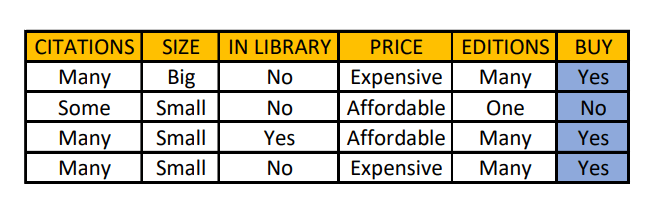### Introduction

In Concept Learning, whenever we search through a predefined space of potential hypotheses in order to find the best fit for training examples in a binary classification problem, then the Find-S Algorithm is one of the first primitive algorithms that can be used to generate the required hypothesis.

In the Find-S Algorithm, we can find a maximally specific hypothesis that fits all the positive examples only in a given data. This algorithm starts with the most specific hypothesis and generalizes it each time the algorithm fails to classify an observed positive training data.

### Algorithm

Here are the steps that need to be followed in order to generate the Hypothesis:

• Initialize the Hypothesis to be in the most specific state.
• For each positive instance:
• For each attribute:
• If the attribute satisfies the constraint, then do nothing
• Else replace the value in the Hypothesis with a more general constraint
• Output the Hypothesis

Note: Most specific hypothesis contains ‘ϕ’ for all the label attributes involved with the data while the most general hypothesis contains ‘?’.

### Inference from the Hypothesis

Let us consider an example. The data given below shows the record of the purchase of various books by a person. Using Find-S Algorithm, we can build a hypothesis and find whether this person will buy a particular book in the future or not.Initially, Hypothesis H = <ϕ,ϕ,ϕ,ϕ,ϕ>

Itemset 1: Label = Yes -> Modify the hypothesis

H = <Many, Big, No, Expensive, Many>

Itemset 2: Label = No-> Ignore the itemset. No change in hypothesis.

H = <Many, Big, No, Expensive, Many>

Itemset 3: Label = Yes -> Modify the hypothesis

H = <Many, ?, ?, ?, Many>

Itemset 4: Label =  Yes -> Modify the hypothesis

H = <Many, ?, ?, ?, Many>

What does this hypothesis infer?

If the given book specifically has many citations and it has many editions, then this person always buys that book. The size of the book, the availability of the book in the library and its prices do not have a specification as such according to the given data.

### Features and Limitations

Some of the key properties of the Find-S Algorithm are:

• It is guaranteed to output the most specific hypothesis that is consistent with positive training examples.
• The final hypothesis will also be consistent with the negative examples provided the correct target concept is obtained in the hypothesis and also provided that the training examples are correct.

The following are the limitations:

• The algorithm finds only one most specific hypothesis. There is no way to find out if any other hypothesis is consistent with the data.
• It ignores the negative examples.
• There is no availability of the option to backtrack the choice of hypothesis found so that the resulting hypothesis can be improved over time.

### Implementation

Let us build a program that computes the hypothesis according to the Find-S algorithm.

				
import java.util.Arrays
val data = arrayOf(
arrayOf("Rainy", "Hot", "High", "False", "No"),
arrayOf("Rainy", "Hot", "High", "True", "No"),
arrayOf("Overcoast", "Hot", "High", "False", "Yes"),
arrayOf("Sunny", "Mild", "High", "False", "Yes"),
arrayOf("Sunny", "Cool", "Normal", "False", "Yes"))
val hypothesis = arrayOfNulls(data.size - 1)
for(i in hypothesis.indices)
{
hypothesis[i] = "ϕ"
}
var flag = true
for (i in data.indices)
{
if(data[i][hypothesis.size] !== "No")
{
if(flag == true)
{
for(j in hypothesis.indices)
{
hypothesis[j] = data[i][j]
}
flag = false
continue
}
for(j in hypothesis.indices)
{
if (hypothesis[j] === "?")
{
continue
}
else if (hypothesis[j] === data[i][j])
{
continue
}
else if(hypothesis[j] !== data[i][j])
{
hypothesis[j] = "?"
continue
}
}
}
}
System.out.print("Hypothesis: " + Arrays.toString(hypothesis))



Output:

				
Hypothesis: [?, ?, ?, False]